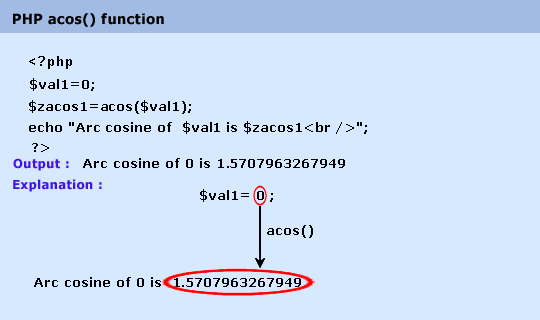# Education is not limited to just classrooms. It can be gained anytime, anywhere... - Ravi Ranjan (M.Tech-NIT)

## PHP : acosh() function

Description

The acosh() function returns the inverse hyperbolic cosine of a number.

Note : This function was not compatible on windows platform before 5.3.0 version.

Version

(PHP 4 and above)

Syntax

acosh(number)

Parameter

Name

Description

Required /
Optional

Type

number

Numeric value.

Required

Float

Return value

The inverse hyperbolic cosine of value.

Value Type : Float.

Pictorial Presentation :Example :

1. <?php
2. \$val1= 0;
3. \$val2 = .4;
4. \$val3 = 1;
5. \$val4 = 2;
6. \$zacos1=acos(\$val1);
7. \$zacos2=acos(\$val2);
8. \$zacos3=acos(\$val3);
9. \$zacos4=acos(\$val4);
10. echo "Arc cosine of  \$val1 is \$abs1<br />";
11. echo "Arc cosine of  \$val2 is \$abs1<br />";
12. echo "Arc cosine of  \$val3 is \$abs1<br />";
13. echo "Arc cosine of  \$val4 is \$abs1<br />";
14. ?>

Output :

Arc cosine of 0 is 1.5707963267949
Arc cosine of 0.4 is 1.1592794807274
Arc cosine of 1 is 0
Arc cosine of 2 is NAN

View the example in the browser

See also

PHP Function Reference